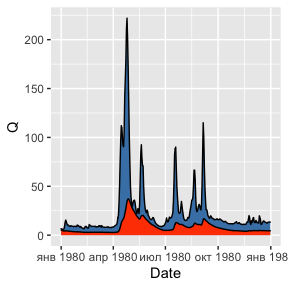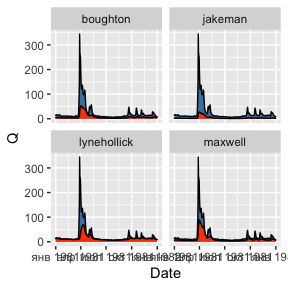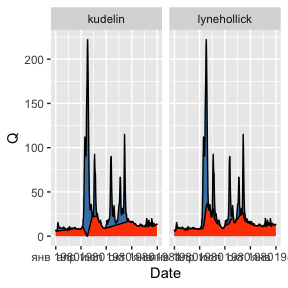# 1. Baseflow filtering

library(grwat)
library(ggplot2)
library(dplyr)
library(tidyr)
library(lubridate)

grwat implements several methods for baseflow filtering, including those by Lyne and Hollick (1979), Chapman (1991), Boughton (1993), Jakeman and Hornberger (1993) and Chapman and Maxwell (1996). The get_baseflow() function does the job:

Qbase = gr_baseflow(spas$Q, method = 'lynehollick', a = 0.925, passes = 3) head(Qbase) #>  3.698598 3.789843 3.876099 3.958334 4.037031 4.112454 Though get_baseflow() needs just a vector of runoff values, it can be applied in a traditional tidyverse pipeline like follows: # Calculate baseflow using Jakeman approach hdata = spas %>% mutate(Qbase = gr_baseflow(Q, method = 'jakeman')) # Visualize for 2020 year ggplot(hdata) + geom_area(aes(Date, Q), fill = 'steelblue', color = 'black') + geom_area(aes(Date, Qbase), fill = 'orangered', color = 'black') + scale_x_date(limits = c(ymd(19800101), ymd(19801231))) #> Warning: Removed 23376 rows containing missing values (position_stack). #> Removed 23376 rows containing missing values (position_stack).Different methods can be compared in a similar way: hdata = spas %>% mutate(lynehollick = gr_baseflow(Q, method = 'lynehollick', a = 0.9), boughton = gr_baseflow(Q, method = 'boughton', k = 0.9), jakeman = gr_baseflow(Q, method = 'jakeman', k = 0.9), maxwell = gr_baseflow(Q, method = 'maxwell', k = 0.9)) %>% pivot_longer(lynehollick:maxwell, names_to = 'Method', values_to = 'Qbase') ggplot(hdata) + geom_area(aes(Date, Q), fill = 'steelblue', color = 'black') + geom_area(aes(Date, Qbase), fill = 'orangered', color = 'black') + scale_x_date(limits = c(ymd(19810101), ymd(19811231))) + facet_wrap(~Method) #> Warning: Removed 93508 rows containing missing values (position_stack). #> Removed 93508 rows containing missing values (position_stack).In case of 100% hydraulic connection between ground waters and river discharge, according to Kudelin (1960) the baseflow is equal to zero level at the point of maximum discharge during the spring flood event. Since extraction of the spring flood requires meteorological data, it cannot be extracted by simple filtering. Instead, you can use the advanced separation method by Rets et al. (2022), which incorporates Kudelin’s approach during the spring flood: p = gr_get_params('center') p$filter = 'kudelin'

hdata = spas %>%
mutate(lynehollick = gr_baseflow(Q, method = 'lynehollick',
a = 0.925, passes = 3),
kudelin = gr_separate(spas, p)\$Qbase) %>%
pivot_longer(lynehollick:kudelin, names_to = 'Method', values_to = 'Qbase')
#> grwat: data frame is correct
#> grwat: parameters list and types are OK

# Visualize for 1980 year
ggplot(hdata) +
geom_area(aes(Date, Q), fill = 'steelblue', color = 'black') +
geom_area(aes(Date, Qbase), fill = 'orangered', color = 'black') +
scale_x_date(limits = c(ymd(19800101), ymd(19801231))) +
facet_wrap(~Method)
#> Warning: Removed 46752 rows containing missing values (position_stack).
#> Removed 46752 rows containing missing values (position_stack).